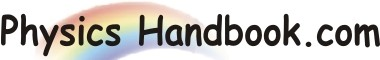HOME TOPICS DEFINITIONS TABLES LAWS INVENTIONS EXPERIMENTS QUIZ VIDEOS
 A B C D E F G H I J K L M N O P Q R S T U V W X Y Z
Beta Decay ( β Decay)
The phenomenon of emission of an electron from a radioactive substance is called β decay.
When there are too many neutrons relative to the number of protons in the nucleus, the repulsive electric force between the protons exceeds the nuclear force and the nucleus becomes unstable. Such a nucleus attains a more stable state when one of its neutrons change into a proton and an electron. As a result, an electron is emitted as β particle.
The proton remains in the nucleus so that the nucleus has lost a neutron and gained a proton i.e. it has become a different nucleus. The β decay can be represented as:
zXA  --->   z+1YA   +    -1e0  +  Eβ
(Parent)   (Daughter)   (β-particle)
Here Eβ is the kinetic energy of the emitted β particle.
For example, carbon 14 decays by β emission to nitrogen according to
6C14  --->   7N14   +    -1e0  +  Eβ
(Parent)   (Daughter)   (β-particle)
Kinetic energy of β particle :
Mass of 6C14  =  14.003242 a.m.u
Mass of 7N14  =  14.003074 a.m.u
Mass defect  =  14.003242 - 14.003074  =  0.000168 a.m.u
Equivalent energy  =  0.000168 x 93.15 MeV  =  0.156 Mev  =  156 Kev